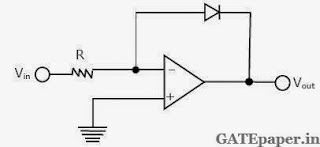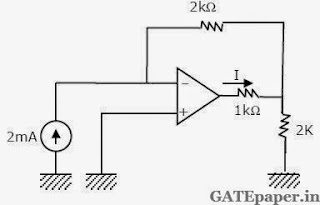### GATE 1992 ECE Analog Circuits (Analog Electronics) - Video Solutions

1. An operational amplifier has an offset voltage of 1 mV and is ideal in all other respects. If this amplifier is used in the circuit shown in figure, the output voltage will be (select the nearest value)a. 1 mV
b. 1 V
c. ± 1 V
d. 0

2. The circuit of figure uses an ideal operational amplifier. For small positive values of Vin, the circuit works asa. Half Wave Rectifier
b. Differentiator
c. Logarithmic amplifier
d. Exponential amplifier

3. Assume that the operational amplifier in figure is ideal. The current I through the 1 kΩ resistor is _____________4. The Two Port Darlington impedance booster of figure uses identical transistors (hie = 1 KΩ, hfe = 100, hre = hoe = 0).Calculate the Z – parameters of the network. Use relevant approximations.

5. The transistors in the differential amplifier shown in figure are identical with hfe = 100 and re = 25 Ω at 1 mA collector current. The circuit has a CMRR of 100.a. What is the differential gain of the circuit?
b. What is the common mode gain of the circuit?
c. If DC voltage of 1010 mV and 990 mV are applied to inputs 1 and 2 respectively with reference to ground, what will be the output voltage Vo?

6. Consider the circuit shown in figure. This circuit uses an ideal operational amplifier.Assuming that the impedance's at nodes A and B do not load the preceding bridge circuit, calculate the output voltage Vo. When (a) RA = RB = RC = RD = 100 Ω and (b) RA = RB = RC = 100 Ω and RD = 120 Ω.